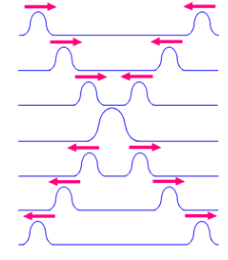# Superposition Formula

## Principle of Superposition

The total current in any part of a linear circuit equals the algebraic sum of the currents produced by each source separately.

For example:

If the response produced by input A is X and that produced by input B is Y, then the response produced by input A+B is X+Y.## According to the Principle of Superposition

It is noted that the net displacement is the algebraic sum of displacements of individual waves.

Consider two waves are travelling alone and the displacement of these two waves can be represented by y1(x, t) and y2(x, t).

When these two waves overlap, the resultant displacement can be given as y(x,t).

y (x, t) = y1(x, t) + y2(x, t)

The resultant wave is calculated by considering the sum of wave functions of travelling waves

y1 = f1(x–vt)

y2 = f2(x–vt)

……….

yn = fn (x–vt)

Hence,

y = f1(x – vt)+ f2(x – vt)+ ……….…+ fn(x – vt)

We can conclude,

$$\begin{array}{l}y=\sum_{i=1}^{n}f_{i}(x-vt)\end{array}$$

Let us consider a wave travelling along a stretched string given by, y1(x, t) = A sin (kx – ωt) and another wave, shifted from the first by a phase φ, given as y2(x, t) = A sin (kx – ωt + φ)

Hence resultant displacement

y (x, t) = A sin (kx – ωt) + A sin (kx – ωt + φ)

This can be written as

$$\begin{array}{l}y(x,t)= {2A cos\frac{1}{2}\phi}sin(kx-wt+\frac{1}{2}\phi )\end{array}$$

We can conclude that the resultant wave is sinusoidal and travels in x direction.

The phase angle is half of the phase difference of the individual waves and the amplitude as

$$\begin{array}{l}2cos\frac{1}{2}\phi\end{array}$$
times the amplitudes of the original waves.